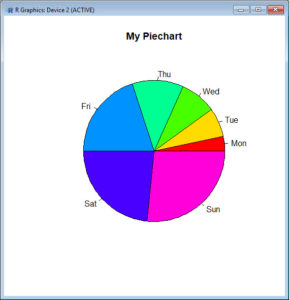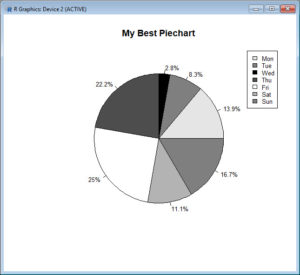In Part 14, let’s see how to create pie charts in R. Let’s create a simple pie chart using the `pie()` command. As always, we set up a vector of numbers and then we plot them.
`B <- c(2, 4, 5, 7, 12, 14, 16)`
Create a simple pie chart.
`pie(B)`Now let’s create a pie chart with a heading, using nice colours, and define our own labels using R’s
`rainbow()` palette. We control the number of colours using `length(B)`.
`pie(B, main="My Piechart", col=rainbow(length(B)), labels=c("Mon","Tue","Wed","Thu","Fri","Sat","Sun"))`Here is a more complex example, using percentages and a legend. We create a vector of data, one for each day of the week
`B <- c(5, 3, 1, 8, 9, 4, 6)`
Set up black, grey and white for clear printing.
`cols <- c("grey90","grey50","black","grey30","white","grey70","grey50")`
Calculate the percentage for each day, using one decimal place.
`percentlabels <- round(100*B/sum(B), 1)`
Add a ‘%’ sign to each percentage value using the `paste()` command.
`pielabels <- paste(percentlabels, "%", sep="")`
What does the `paste()` command do?
`pie(B, main="My Best Piechart", col=cols, labels=pielabels, cex=0.8)`
Create a legend at the top-right.
`legend("topright", c("Mon","Tue","Wed","Thu","Fri","Sat","Sun"), cex=0.8, fill=cols)`OK. Now let’s create a pie chart from a data frame and include sample sizes. First create a table of counts of cylinder numbers from the `mtcars` dataset.
```cyltable <- table(mtcars\$cyl) cyltable```We have eleven cars with four cylinders, seven cars with six cylinders, and fourteen cars with eight cylinders.
Now we create labels.
`labs <- paste("(",names(cyltable),")", "\n", cyltable, sep="")`
Now we plot.
`pie(cyltable, labels = labs, col = c("red", "yellow", "blue"), main="Pie chart of cylinder numbers\n with sample sizes")`That wasn’t so hard! In Blog 15 we will look at further plotting techniques in R.
See you later!
David

#### Annex: R codes used

```# Create a vector with numbers.
B <- c(2, 4, 5, 7, 12, 14, 16)

# Create a simple pie chart.
pie(B)

# Create a pie chart with rainbow colours.
pie(B, main="My Piechart", col=rainbow(length(B)), labels=c("Mon","Tue","Wed","Thu","Fri","Sat","Sun"))

# Create a vector with numbers.
B <- c(5, 3, 1, 8, 9, 4, 6)

# Set up black, grey and white for clear printing.
cols <- c("grey90","grey50","black","grey30","white","grey70","grey50")

# Calculate the percentage for each day, using one decimal place.
percentlabels <- round(100*B/sum(B), 1)

# Add a '%' sign to each percentage value using the paste command.
pielabels <- paste(percentlabels, "%", sep="")

# Create a pie chart.
pie(B, main="My Best Piechart", col=cols, labels=pielabels, cex=0.8)

# Add a legend at the right to existing pie chart.
legend("topright", c("Mon","Tue","Wed","Thu","Fri","Sat","Sun"), cex=0.8, fill=cols)

# Create a table of counts of cylinder numbers from the mtcars dataset.
cyltable <- table(mtcars\$cyl)
cyltable

# Create labels.
labs <- paste("(",names(cyltable),")", "\n", cyltable, sep="")

# Create a pie chart.
pie(cyltable, labels = labs, col = c("red", "yellow", "blue"), main="Pie chart of cylinder numbers\n with sample sizes")
```